Next: Examples Up: Implementation Previous: General principle   Contents

The variable table

Assume now that you have chosen a mixed bisection in which the bisection is applied on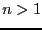variables. The procedure will choose the bisected variables using, for example, the smear function. But in some cases it may be interesting to guide the bisection: for example if we know that subsets of the variables have a strong influence on the extremal value of the optimum function it may be interesting to indicate that as soon as the smear function has led to bisecting one variable in a given subset it may be good to bisect also the other variables in the subset. For example consider the following functions: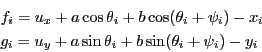where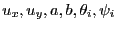are unknowns and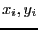are given. Consider now the optimum function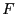: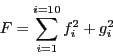which has 24 unknowns. But clearly each subset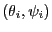has a strong influence on the minimum of. Hence if one of the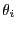is bisected it may be interesting to bisect also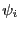. This may be done by setting the flag ALIAS_Guide_Bisection to 1 and using the variables table: for a problem with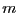unknowns the variables table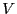is an array of size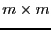and a 1 in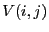indicates that if the variable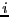is bisected then the variable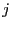should be also bisected. In ALIAS the variables table is implemented under the name ALIAS_Bisecting_Table. It is the responsibility of the user to clear this array and update it as in the following example:

Resize(ALIAS_Bisecting_Table,24,24);
Clear(ALIAS_Bisecting_Table);
ALIAS_Bisecting_Table(1,2)=1;
ALIAS_Bisecting_Table(2,1)=1;
ALIAS_Bisecting_Table(3,4)=1;
ALIAS_Bisecting_Table(4,3)=1;Next: Examples Up: Implementation Previous: General principle   Contents
Jean-Pierre Merlet 2012-12-20